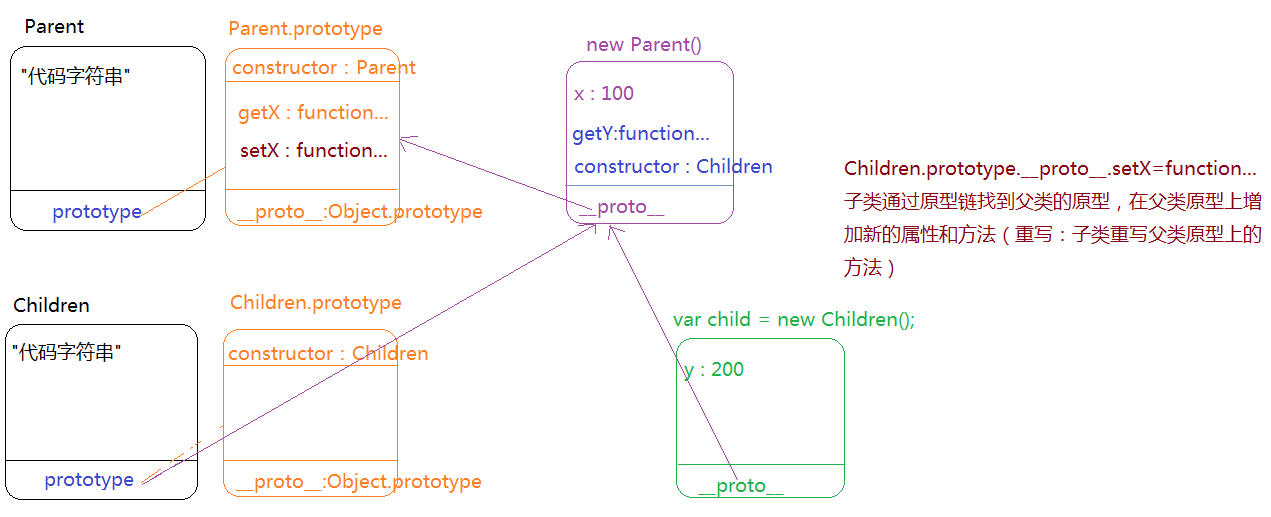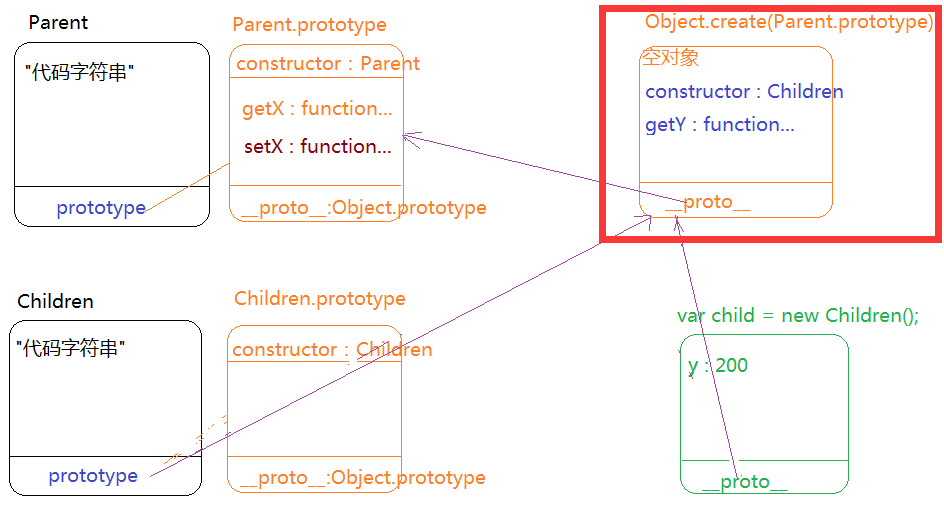# 关于JS中面向对象的理解

### 面向对象编程(OOP)

`封装`

`多态`

[ 重载 ]

``public void sum(int num1,int num2){//=>CODE} public void sum(int num1){//=>CODE} public void sum(int num1,string str){//=>CODE} sum(12,23)sum(12)sum(12,'珠峰')``

JS中没有类似于后台严格意义上的重载：JS中如果方法名相同了，最后只能保留一个（和实参没关系的）
JS中的重载：同一个方法，通过传递实参的不同（arguments）我们完成不同的功能，我们把这个也可以理解为重载

``function sum(num1,num2){return num1+num2;}function sum(){var ary=[].slice.call(argumnets);return eval(ary.join('+'));}sum(10,20);sum(10,20,30);//=>不管哪一次执行的都是第二个SUM``

### 类的继承

`原型继承`

Children.prototype = new Parent();

[细节]
1、我们首先让子类的原型指向父类的实例，然后再向子类原型上扩展方法，防止提前增加方法，等原型重新指向后，之前在子类原型上扩展的方法都没用了（子类原型已经指向新的空间地址了）
2、让子类原型重新指向父类实例，子类原型上原有的constructor就没有了，为了保证构造函数的完整性，我们最好给子类的原型重新手动设置constructor属性值：`Children.prototype.constructor = Children;`

[ 原理 ]

[ 特征 ]

``function Parent() {this.x = 100;}Parent.prototype.getX = function () {console.log(this.x);}; function Children() {this.y = 200;}Children.prototype = new Parent();//=>最好在扩展子类原型方法之前完成Children.prototype.constructor = Children;Children.prototype.getY = function () {console.log(this.y);}; var child = new Children();```call继承`

``function Parent() {this.x = 100;}Parent.prototype.getX = function () {console.log(this.x);};function Children() {//=>this:child 子类的实例Parent.call(this);//=>让Parent执行,方法中的THIS依然是子类的实例（在父类构造体中写的 THIS.XXX=XXX 都相当于在给子类的实例增加一些私有的属性和方法）this.y = 200;}var child = new Children();``

[ 原理 ]

[ 细节 ]

`寄生组合继承`

Object.create([obj])：创建一个空对象（实例），把[obj]作为新创建对象的原型

``var obj={name:'珠峰培训'};var newObj=Object.create(obj);newObj.__proto__===obj``

``function Parent() {this.x = 100;}Parent.prototype.getX = function () {console.log(this.x);};function Children() {Parent.call(this);this.y = 200;}Children.prototype = Object.create(Parent.prototype);Children.prototype.constructor = Children;Children.prototype.getY = function () {console.log(this.y);};````Object.myCreate = function myCreate(obj) {var Fn = new Function();Fn.prototype = obj;return new Fn();};``

### ES6中的类及继承

`创建类`

``class Fn {constructor(){//=>constructor:Fn//=>这里面的 this.xxx=xxx 是给当前实例设置的私有属性}//=>这里设置的方法都放在Fn.prototype上（给实例提供的公有属性方法）xxx(){}... //=>这写属性和方法都是把Fn当做普通对象设置的私有属性和方法，和实例没有任何的关系static xxx(){}...}var f = new Fn();``

`继承`

``class A {constructor() {this.x = 100;} getX() {console.log(this.x);}} class B extends A {constructor() {super();//=>CALL继承 进来第一句话必须写上super()this.y = 200;} getY() {console.log(this.y);}} var b = new B();``